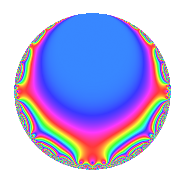# Properties

 Label 4018.2.a.tLevel 4018 Weight 2 Character orbit 4018.a Self dual Yes Analytic conductor 32.084 Analytic rank 1 Dimension 2 CM No Inner twists 1

# Related objects

## Newspace parameters

 Level: $$N$$ = $$4018 = 2 \cdot 7^{2} \cdot 41$$ Weight: $$k$$ = $$2$$ Character orbit: $$[\chi]$$ = 4018.a (trivial)

## Newform invariants

 Self dual: Yes Analytic conductor: $$32.0838915322$$ Analytic rank: $$1$$ Dimension: $$2$$ Coefficient field: $$\Q(\sqrt{5})$$ Coefficient ring: $$\Z[a_1, \ldots, a_{5}]$$ Coefficient ring index: $$2$$ Fricke sign: $$1$$ Sato-Tate group: $\mathrm{SU}(2)$

## $q$-expansion

Coefficients of the $$q$$-expansion are expressed in terms of $$\beta = \sqrt{5}$$. We also show the integral $$q$$-expansion of the trace form.

 $$f(q)$$ $$=$$ $$q$$ $$- q^{2}$$ $$- q^{3}$$ $$+ q^{4}$$ $$+ \beta q^{5}$$ $$+ q^{6}$$ $$- q^{8}$$ $$-2 q^{9}$$ $$+O(q^{10})$$ $$q$$ $$- q^{2}$$ $$- q^{3}$$ $$+ q^{4}$$ $$+ \beta q^{5}$$ $$+ q^{6}$$ $$- q^{8}$$ $$-2 q^{9}$$ $$-\beta q^{10}$$ $$+ 2 \beta q^{11}$$ $$- q^{12}$$ $$+ ( -2 - 2 \beta ) q^{13}$$ $$-\beta q^{15}$$ $$+ q^{16}$$ $$-3 q^{17}$$ $$+ 2 q^{18}$$ $$+ 4 q^{19}$$ $$+ \beta q^{20}$$ $$-2 \beta q^{22}$$ $$+ ( 4 - 2 \beta ) q^{23}$$ $$+ q^{24}$$ $$+ ( 2 + 2 \beta ) q^{26}$$ $$+ 5 q^{27}$$ $$+ ( 4 + \beta ) q^{29}$$ $$+ \beta q^{30}$$ $$+ ( -4 + \beta ) q^{31}$$ $$- q^{32}$$ $$-2 \beta q^{33}$$ $$+ 3 q^{34}$$ $$-2 q^{36}$$ $$+ ( -4 - 2 \beta ) q^{37}$$ $$-4 q^{38}$$ $$+ ( 2 + 2 \beta ) q^{39}$$ $$-\beta q^{40}$$ $$+ q^{41}$$ $$+ 9 q^{43}$$ $$+ 2 \beta q^{44}$$ $$-2 \beta q^{45}$$ $$+ ( -4 + 2 \beta ) q^{46}$$ $$+ ( 4 - 4 \beta ) q^{47}$$ $$- q^{48}$$ $$+ 3 q^{51}$$ $$+ ( -2 - 2 \beta ) q^{52}$$ $$-3 \beta q^{53}$$ $$-5 q^{54}$$ $$+ 10 q^{55}$$ $$-4 q^{57}$$ $$+ ( -4 - \beta ) q^{58}$$ $$+ ( -8 + 2 \beta ) q^{59}$$ $$-\beta q^{60}$$ $$+ ( -8 + 3 \beta ) q^{61}$$ $$+ ( 4 - \beta ) q^{62}$$ $$+ q^{64}$$ $$+ ( -10 - 2 \beta ) q^{65}$$ $$+ 2 \beta q^{66}$$ $$+ ( 2 - 6 \beta ) q^{67}$$ $$-3 q^{68}$$ $$+ ( -4 + 2 \beta ) q^{69}$$ $$+ \beta q^{71}$$ $$+ 2 q^{72}$$ $$-6 q^{73}$$ $$+ ( 4 + 2 \beta ) q^{74}$$ $$+ 4 q^{76}$$ $$+ ( -2 - 2 \beta ) q^{78}$$ $$+ ( 4 - 3 \beta ) q^{79}$$ $$+ \beta q^{80}$$ $$+ q^{81}$$ $$- q^{82}$$ $$+ ( -6 + 4 \beta ) q^{83}$$ $$-3 \beta q^{85}$$ $$-9 q^{86}$$ $$+ ( -4 - \beta ) q^{87}$$ $$-2 \beta q^{88}$$ $$+ 3 q^{89}$$ $$+ 2 \beta q^{90}$$ $$+ ( 4 - 2 \beta ) q^{92}$$ $$+ ( 4 - \beta ) q^{93}$$ $$+ ( -4 + 4 \beta ) q^{94}$$ $$+ 4 \beta q^{95}$$ $$+ q^{96}$$ $$- q^{97}$$ $$-4 \beta q^{99}$$ $$+O(q^{100})$$ $$\operatorname{Tr}(f)(q)$$ $$=$$ $$2q$$ $$\mathstrut -\mathstrut 2q^{2}$$ $$\mathstrut -\mathstrut 2q^{3}$$ $$\mathstrut +\mathstrut 2q^{4}$$ $$\mathstrut +\mathstrut 2q^{6}$$ $$\mathstrut -\mathstrut 2q^{8}$$ $$\mathstrut -\mathstrut 4q^{9}$$ $$\mathstrut +\mathstrut O(q^{10})$$ $$2q$$ $$\mathstrut -\mathstrut 2q^{2}$$ $$\mathstrut -\mathstrut 2q^{3}$$ $$\mathstrut +\mathstrut 2q^{4}$$ $$\mathstrut +\mathstrut 2q^{6}$$ $$\mathstrut -\mathstrut 2q^{8}$$ $$\mathstrut -\mathstrut 4q^{9}$$ $$\mathstrut -\mathstrut 2q^{12}$$ $$\mathstrut -\mathstrut 4q^{13}$$ $$\mathstrut +\mathstrut 2q^{16}$$ $$\mathstrut -\mathstrut 6q^{17}$$ $$\mathstrut +\mathstrut 4q^{18}$$ $$\mathstrut +\mathstrut 8q^{19}$$ $$\mathstrut +\mathstrut 8q^{23}$$ $$\mathstrut +\mathstrut 2q^{24}$$ $$\mathstrut +\mathstrut 4q^{26}$$ $$\mathstrut +\mathstrut 10q^{27}$$ $$\mathstrut +\mathstrut 8q^{29}$$ $$\mathstrut -\mathstrut 8q^{31}$$ $$\mathstrut -\mathstrut 2q^{32}$$ $$\mathstrut +\mathstrut 6q^{34}$$ $$\mathstrut -\mathstrut 4q^{36}$$ $$\mathstrut -\mathstrut 8q^{37}$$ $$\mathstrut -\mathstrut 8q^{38}$$ $$\mathstrut +\mathstrut 4q^{39}$$ $$\mathstrut +\mathstrut 2q^{41}$$ $$\mathstrut +\mathstrut 18q^{43}$$ $$\mathstrut -\mathstrut 8q^{46}$$ $$\mathstrut +\mathstrut 8q^{47}$$ $$\mathstrut -\mathstrut 2q^{48}$$ $$\mathstrut +\mathstrut 6q^{51}$$ $$\mathstrut -\mathstrut 4q^{52}$$ $$\mathstrut -\mathstrut 10q^{54}$$ $$\mathstrut +\mathstrut 20q^{55}$$ $$\mathstrut -\mathstrut 8q^{57}$$ $$\mathstrut -\mathstrut 8q^{58}$$ $$\mathstrut -\mathstrut 16q^{59}$$ $$\mathstrut -\mathstrut 16q^{61}$$ $$\mathstrut +\mathstrut 8q^{62}$$ $$\mathstrut +\mathstrut 2q^{64}$$ $$\mathstrut -\mathstrut 20q^{65}$$ $$\mathstrut +\mathstrut 4q^{67}$$ $$\mathstrut -\mathstrut 6q^{68}$$ $$\mathstrut -\mathstrut 8q^{69}$$ $$\mathstrut +\mathstrut 4q^{72}$$ $$\mathstrut -\mathstrut 12q^{73}$$ $$\mathstrut +\mathstrut 8q^{74}$$ $$\mathstrut +\mathstrut 8q^{76}$$ $$\mathstrut -\mathstrut 4q^{78}$$ $$\mathstrut +\mathstrut 8q^{79}$$ $$\mathstrut +\mathstrut 2q^{81}$$ $$\mathstrut -\mathstrut 2q^{82}$$ $$\mathstrut -\mathstrut 12q^{83}$$ $$\mathstrut -\mathstrut 18q^{86}$$ $$\mathstrut -\mathstrut 8q^{87}$$ $$\mathstrut +\mathstrut 6q^{89}$$ $$\mathstrut +\mathstrut 8q^{92}$$ $$\mathstrut +\mathstrut 8q^{93}$$ $$\mathstrut -\mathstrut 8q^{94}$$ $$\mathstrut +\mathstrut 2q^{96}$$ $$\mathstrut -\mathstrut 2q^{97}$$ $$\mathstrut +\mathstrut O(q^{100})$$

## Embeddings

For each embedding $$\iota_m$$ of the coefficient field, the values $$\iota_m(a_n)$$ are shown below.

For more information on an embedded modular form you can click on its label.

Label $$\iota_m(\nu)$$ $$a_{2}$$ $$a_{3}$$ $$a_{4}$$ $$a_{5}$$ $$a_{6}$$ $$a_{7}$$ $$a_{8}$$ $$a_{9}$$ $$a_{10}$$
1.1
 −0.618034 1.61803
−1.00000 −1.00000 1.00000 −2.23607 1.00000 0 −1.00000 −2.00000 2.23607
1.2 −1.00000 −1.00000 1.00000 2.23607 1.00000 0 −1.00000 −2.00000 −2.23607
 $$n$$: e.g. 2-40 or 990-1000 Significant digits: Format: Complex embeddings Normalized embeddings Satake parameters Satake angles

## Inner twists

This newform does not admit any (nontrivial) inner twists.

## Atkin-Lehner signs

$$p$$ Sign
$$2$$ $$1$$
$$7$$ $$-1$$
$$41$$ $$-1$$

## Hecke kernels

This newform can be constructed as the intersection of the kernels of the following linear operators acting on $$S_{2}^{\mathrm{new}}(\Gamma_0(4018))$$:

 $$T_{3}$$ $$\mathstrut +\mathstrut 1$$ $$T_{5}^{2}$$ $$\mathstrut -\mathstrut 5$$ $$T_{11}^{2}$$ $$\mathstrut -\mathstrut 20$$